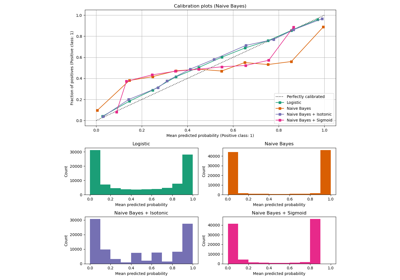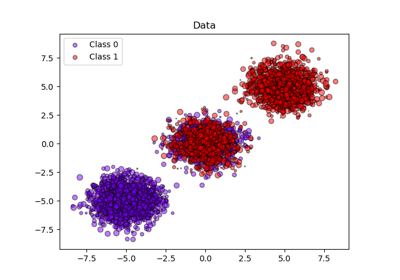# sklearn.metrics.brier_score_loss¶

sklearn.metrics.brier_score_loss(y_true, y_prob, *, sample_weight=None, pos_label=None)[source]

Compute the Brier score loss.

The smaller the Brier score loss, the better, hence the naming with “loss”. The Brier score measures the mean squared difference between the predicted probability and the actual outcome. The Brier score always takes on a value between zero and one, since this is the largest possible difference between a predicted probability (which must be between zero and one) and the actual outcome (which can take on values of only 0 and 1). It can be decomposed as the sum of refinement loss and calibration loss.

The Brier score is appropriate for binary and categorical outcomes that can be structured as true or false, but is inappropriate for ordinal variables which can take on three or more values (this is because the Brier score assumes that all possible outcomes are equivalently “distant” from one another). Which label is considered to be the positive label is controlled via the parameter pos_label, which defaults to the greater label unless y_true is all 0 or all -1, in which case pos_label defaults to 1.

Read more in the User Guide.

Parameters:
y_truearray of shape (n_samples,)

True targets.

y_probarray of shape (n_samples,)

Probabilities of the positive class.

sample_weightarray-like of shape (n_samples,), default=None

Sample weights.

pos_labelint or str, default=None

Label of the positive class. pos_label will be inferred in the following manner:

• if y_true in {-1, 1} or {0, 1}, pos_label defaults to 1;

• else if y_true contains string, an error will be raised and pos_label should be explicitly specified;

• otherwise, pos_label defaults to the greater label, i.e. np.unique(y_true)[-1].

Returns:
scorefloat

Brier score loss.

References

Examples

>>> import numpy as np
>>> from sklearn.metrics import brier_score_loss
>>> y_true = np.array([0, 1, 1, 0])
>>> y_true_categorical = np.array(["spam", "ham", "ham", "spam"])
>>> y_prob = np.array([0.1, 0.9, 0.8, 0.3])
>>> brier_score_loss(y_true, y_prob)
0.037...
>>> brier_score_loss(y_true, 1-y_prob, pos_label=0)
0.037...
>>> brier_score_loss(y_true_categorical, y_prob, pos_label="ham")
0.037...
>>> brier_score_loss(y_true, np.array(y_prob) > 0.5)
0.0


## Examples using sklearn.metrics.brier_score_loss¶Probability Calibration curves

Probability Calibration curvesProbability calibration of classifiers

Probability calibration of classifiers